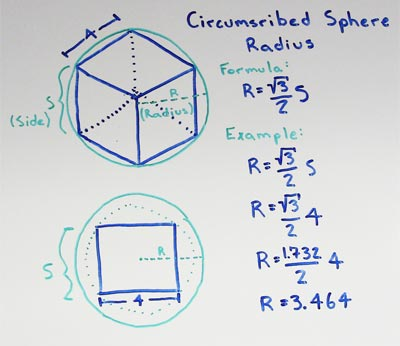# Volume of Cube

A cube with a side length (S) of 6 has a circumscribed sphere with a radius (R) of 5.196.

for cubes with a side length S find the radius R of the circumscribed sphere

• R = S * (√3/2)

substitute the side length S with the measured value, in this example lets use a side length of 6

• R = 6 * (√3/2)

multiply the side length by half the square root of three

• R = 5.196
•Given a cube with a side length S the radius (R) of a sphere that circumscribes it will be equal to the Side length multiplied by half the square root of 3.

Half the square root of 3 is approximately 0.8660

### Formulas

What is the formula for the volume of a Cube?

V = S3

What is the formula for the volume of a Rectangular Prism?

V = L x W x H

### Other Formulae

for cubes with side length S

Cube Surface Area

6S2
Cube Face Diagonal
(√2)S
Cube Space Diagonal
(√3)S
S/2
All formulae...